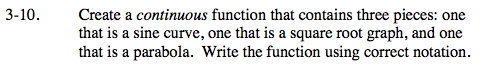### Home > CALC > Chapter Ch3 > Lesson 3.1.1 > Problem3-10

3-10.To be continuous at a point,
1) the limit must exist (agree from the left and the right)
2) the value must exist (be finite)
3) the limit must agree with the value.

$\text{You could use the parent graphs: }y=\text{sin}x,\ y=\sqrt{x},$

$\text{ and } y = x^{2} \text{or a transformation of any of them.}$

If you choose to use the parent graphs, find the location of points that two of them share:

For example:

$\text{sin}x=\sqrt{x}$

$x=\underline{ \ \ \ \ \ \ \ \ \ }$

$\sqrt{x}=x^{2}$

$x=\underline{ \ \ \ \ \ \ \ \ \ }\text{ and }\underline{ \ \ \ \ \ \ \ \ \ }$

$x^{2}=\text{sin}x$

$x=\underline{ \ \ \ \ \ \ \ \ \ }\text{ and }\underline{ \ \ \ \ \ \ \ \ \ }$

These x-values will give you possible boundary points for your piecewise function.

Arrange the three functions in a way that will make a continuous piecewise graph, meeting at boundary points.

Use the eTool below to create the piecewise function.
Click on the link to the right to view the full version of the eTool. Calc 3-10 HW eTool